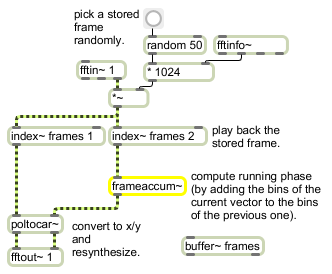# frameaccum~

Compute "running phase" of successive phase deviation frames

## Description

frameaccum~ computes a running phase by keeping a sum of the values in each position of its incoming signal vectors. When used inside a pfft~ object, it can keep a running phase of the FFT because the FFT size is equal to the signal vector size.

## Arguments

Name Type Opt Description
phasewrap-flag (0 or nonzero) int opt A non-zero integer argument will cause the accumulated values to be wrapped between -π and π. This optional feature is to reduce roundoff error when using frameaccum~ to accumulate phase values. When the argument is set to one. frameaccum~ object performs a phase warping (like the phasewrap~ object). It is more efficient to use the non-zero argument than to use a combination of frameaccum~ and phasewrap~ objects.

## Messages

 signal The input to be accumulated.

## Output

signal: For each signal vector, the first sample of its output will be the sum of all of the first samples in each signal vector it has received, the second sample of its output will be the sum of all the second samples in each signal vector, and so on.

## Examplesframeaccum~ computes the running phase between frames of spectral data